# 专注，勤学，慎思。戒骄戒躁，谦虚谨慎

just do it

## MySQL分区表

MySQL分区表对分区字段的限制

CREATE TABLE t1 (
col1 INT NOT NULL,
col2 DATE NOT NULL,
col3 INT NOT NULL,
col4 INT NOT NULL,
UNIQUE KEY (col1, col2)
)
PARTITION BY HASH(col3)
PARTITIONS 4;

CREATE TABLE t2 (
col1 INT NOT NULL,
col2 DATE NOT NULL,
col3 INT NOT NULL,
col4 INT NOT NULL,
PRIMARY KEY (col1),
UNIQUE KEY (col3)
)
PARTITION BY HASH(col1 + col3)
PARTITIONS 4;

CREATE TABLE t4 (
col1 INT NOT NULL,
col2 INT NOT NULL,
col3 INT NOT NULL,
col4 INT NOT NULL,
UNIQUE KEY (col1, col3),
UNIQUE KEY (col2, col4)、
);

MySQL是局部分区，意思是一个分区中，包含分区的数据和其对应的索引，而不是索引是一个索引统一存放在一个地方，仅分区数据这种方式。

range分区,分区字段必须是整型或者转换为整型

CREATE TABLE test_range_partition(
id INT auto_increment,
createdate DATETIME,
primary key (id,createdate)
)
PARTITION BY RANGE (TO_DAYS(createdate) ) (
PARTITION p201801 VALUES LESS THAN ( TO_DAYS('20180201') ),
PARTITION p201802 VALUES LESS THAN ( TO_DAYS('20180301') ),
PARTITION p201803 VALUES LESS THAN ( TO_DAYS('20180401') ),
PARTITION p201804 VALUES LESS THAN ( TO_DAYS('20180501') ),
PARTITION p201805 VALUES LESS THAN ( TO_DAYS('20180601') ),
PARTITION p201806 VALUES LESS THAN ( TO_DAYS('20180701') ),
PARTITION p201807 VALUES LESS THAN ( TO_DAYS('20180801') ),
PARTITION p201808 VALUES LESS THAN ( TO_DAYS('20180901') ),
PARTITION p201809 VALUES LESS THAN ( TO_DAYS('20181001') ),
PARTITION p201810 VALUES LESS THAN ( TO_DAYS('20181101') ),
PARTITION p201811 VALUES LESS THAN ( TO_DAYS('20181201') ),
PARTITION p201812 VALUES LESS THAN ( TO_DAYS('20190101') )
);

insert into test_range_partition (createdate) values ('20180105');
insert into test_range_partition (createdate) values ('20180205');
insert into test_range_partition (createdate) values ('20180206');
insert into test_range_partition (createdate) values ('20180305');
insert into test_range_partition (createdate) values ('20180405');
insert into test_range_partition (createdate) values ('20180505');
insert into test_range_partition (createdate) values ('20180605');
insert into test_range_partition (createdate) values ('20180705');
insert into test_range_partition (createdate) values ('20180805');
insert into test_range_partition (createdate) values ('20180905');
insert into test_range_partition (createdate) values ('20181005');
insert into test_range_partition (createdate) values ('20181105');

select
table_schema,
table_name,
partition_name,
partition_ordinal_position,
partition_method,
partition_expression,
table_rows
from information_schema.PARTITIONS where table_schema = 'db01' and table_name = 'test_range_partition';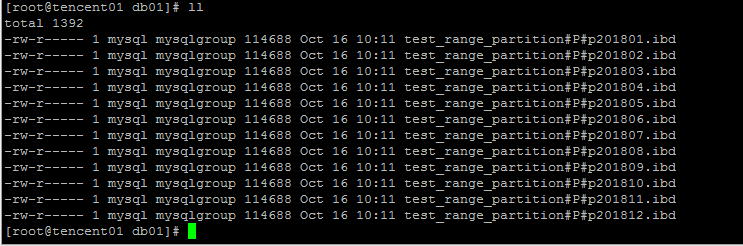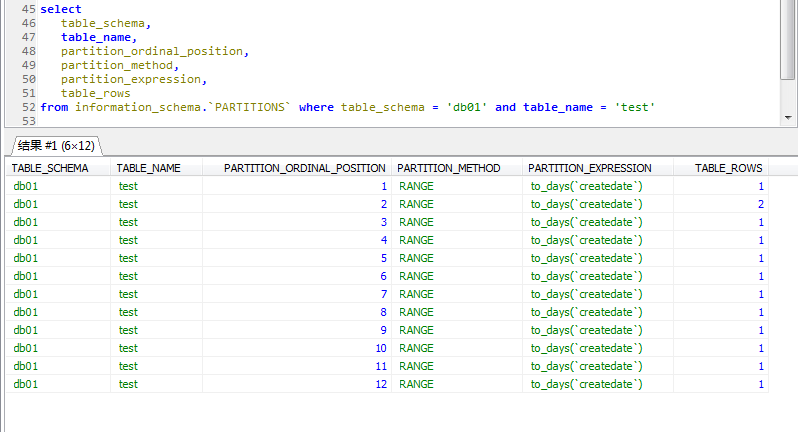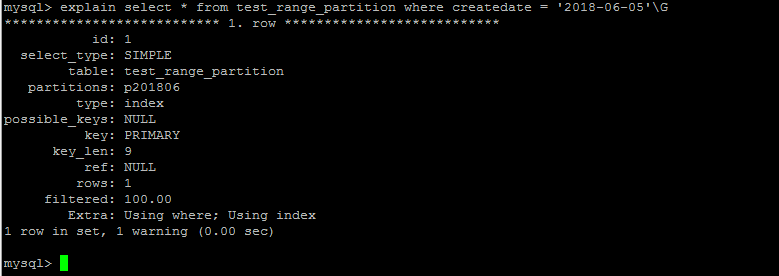ALTER TABLE test ADD PARTITION (PARTITION p201902 VALUES LESS THAN ( TO_DAYS('20190301') ));
ALTER TABLE test DROP PARTITION p20180201;

List分区，分区字段必须是整型或者转换为整型

create table test_list_partiotion
(
id int auto_increment,
data_type tinyint,
primary key(id,data_type)
)partition by list(data_type)
(
partition p0 values in (0,1,2,3,4,5,6),
partition p1 values in (7,8,9,10,11,12),
partition p2 values in (13,14,15,16,17)
);

Hash分区，分区字段必须是整型或者转换为整型
Hash分区可以将数据均匀地分不到预先定义的分区中，使得各个分区的数据量分布基本上一致。同样，分区字段必须是整型或者转换为整型

drop table test_hash_partiotion;
create table test_hash_partiotion
(
id int auto_increment,
create_date datetime,
primary key(id,create_date)
)partition by hash(year(create_date)) partitions 10;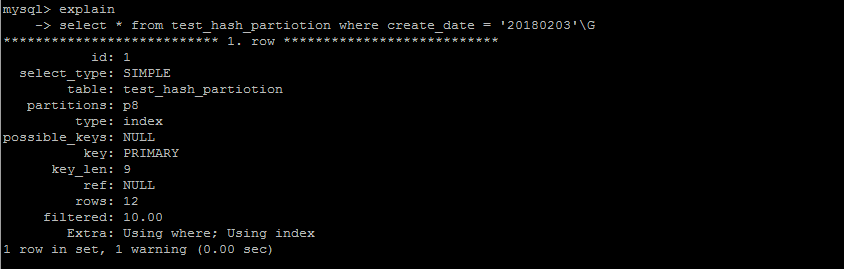hash分区在查询优化方面，无法优化范围查询，因为无法确定一个某个字段经过hash计算之后究竟分布了在哪个分区之中。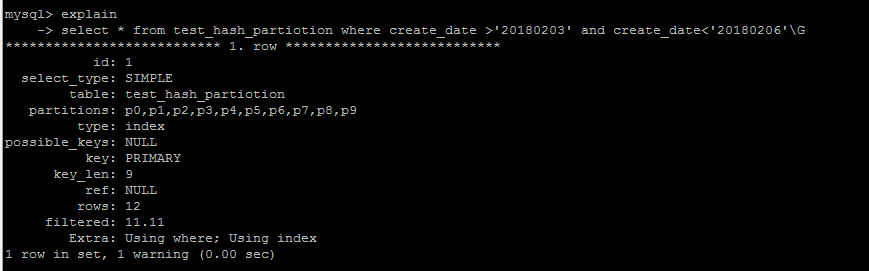Key分区，分区字段必须是整型或者转换为整型

create table test_key_partiotion
(
id int auto_increment,
create_date datetime,
primary key(id,create_date)
)partition by key(create_date) partitions 10;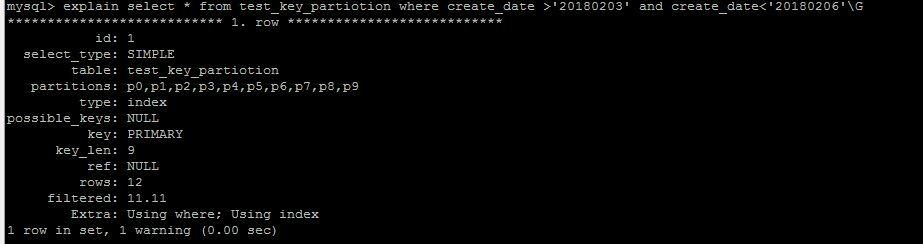column 分区

create table test_column_partiotion
(
id int auto_increment,
data_type datetime,
primary key(id,data_type)
)partition by range columns(data_type) (
partition p0 values less than ('20180101'),
partition p1 values less than ('20180201'),
partition p2 values less than ('20180301'),
partition p3 values less than ('20180401'),
partition p4 values less than ('20180501'),
partition p5 values less than ('20180601'),
partition p6 values less than ('20180701'),
partition p7 values less than ('20180801')
);

posted on 2018-10-16 10:58  MSSQL123  阅读(32805)  评论(1编辑  收藏  举报